Latest Banking jobs   »

# Quantitative Aptitude Quiz For LIC ADO Mains 2023- 22nd March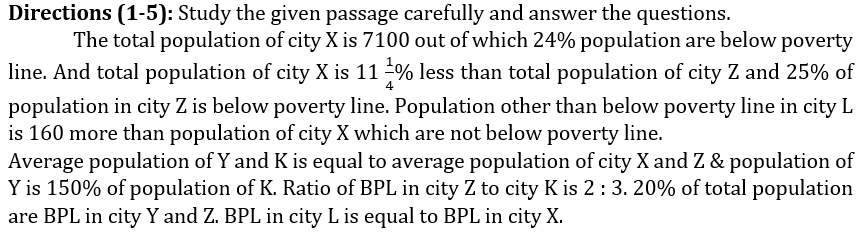Q1. Average BPL population in city X and Y is how much more/less than average BPL population in city K and L ?
(a) 794
(b) 824
(c) 848
(d) 764
(e) None of these
Q2. Average population in city K and L is what percent of total population in city Z ?
(a) 93%
(b) 73%
(c) 83.125%
(d) 87.50%
(e) 78.625%

Q3. In another city ‘A’, BPL population is half of the population other than BPL in city K which is 25% of total population. Then find total population in city A ?
(a) 6820
(b) 6080
(c) 6240
(d) None of these
(e) 6040

Q4. What is the average BPL population in all the cities ?
(a) 1924
(b) None of these
(c) 1884
(d) 1724
(e) 1964

Q5. What is the ratio of BPL population in city Y to city L ?
(a) 426 : 353
(b) 353 : 426
(c) 351 : 425
(d) None of these
(e) 353 : 428
Direction (6 – 10): Line graph shows marked up percentage of four articles above cost price and table shows marked price of these four articles. Read the data carefully and answer the questions.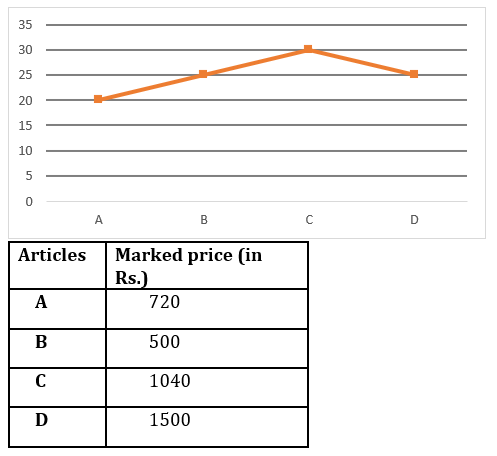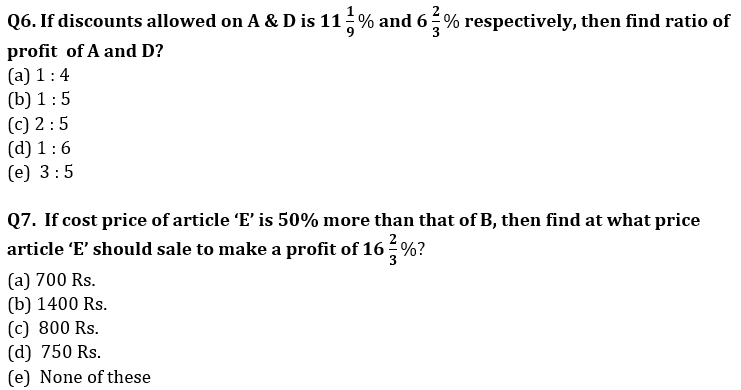Q8. If discount (in %) given on article B & D is in the ratio of 1 : 3 and ratio of profit on article B & loss on article D is same (in Rs) , then find discount allowed on article D?
(a) 18%
(b) 12%
(c) 30%
(d) 15 %
(e) 24%

Q9. Cost price of A is what percent less than average cost price of B, C & D ?
(a) 12%
(b) 10%
(c) 15%
(d) 25%
(e) 20%

Q10. Discount allowed on article C is 15% and selling price of article ‘X’ is Rs. 316 more than that of C. If profit made on ‘X’ is 20%, then find difference between cost price of D & X?
(a) 300 Rs.
(b) 600 Rs.
(c) 400 Rs.
(d) 200 Rs.
(e) 100 Rs.
Directions (11-15): Study the passage given below and answer the following questions.
There are 3 cities – Delhi, Dhaka and Bangkok. Total distance between Delhi – Dhaka, Dhaka – Bangkok and Delhi – Bangkok is 19800 km. Distance between Dhaka – Bangkok is 80% of the distance between Delhi – Bangkok and ratio of distance between Delhi – Dhaka to distance between Bangkok – Dhaka is 27 : 32.
Fares of 3 different flight operators (A, B & C) on these 3 routes (Delhi – Dhaka, Dhaka – Bangkok and Delhi – Bangkok) are –
Delhi – Dhaka: Fare of C is 25% more than that of B and fare of A is Rs.2700 less than that of B. Ratio of fare of A to that of C is 3 : 5.
Delhi – Bangkok: Average fare of A & B is Rs.20000 and fare of C is Rs.8000 more than average fare of A & B. Average fare of B & C is Rs.26000.
Dhaka – Bangkok: Fare of B is Rs.16000 and ratio of fare of A to that of B to that of C is 6 : 5 : 8.

Q11. If Veer wants to travel from Delhi – Dhaka and then Dhaka – Bangkok without changing flight operator, then which flight operator would be the cheapest option among A, B & C for Veer.
(a) A
(b) B
(c) C
(d) Either A or B
(e) Either A or C

Q12. If Deepak is travelling from Dhaka – Bangkok and Bangkok – Delhi from C, then find the amount paid by Deepak per km for the entire trip.
(a) Rs. 3.11/km
(b) Rs. 3.98/km
(c) Rs. 3.42/km
(d) Rs. 3.62/km
(e) Rs. 3.01/km

Q13. For which of the following trip, the fare will be maximum?
(a) A, Delhi – Bangkok
(b) C, Delhi – Dhaka
(c) B, Dhaka – Bangkok
(d) A, Dhaka – Bangkok
(e) B, Delhi – Dhaka

Q14. On Dhaka – Bangkok route, which flight operator is charging lowest fare per km?
(a) A
(b) B
(c) C
(d) Either B or C
(e) Cannot be determined.
Q15. Find average fare of A, B & C on Delhi – Dhaka route.
(a) Rs.12000
(b) Rs.11500
(c) Rs.10400
(d) Rs.11200
(e) Rs.10800

Solutions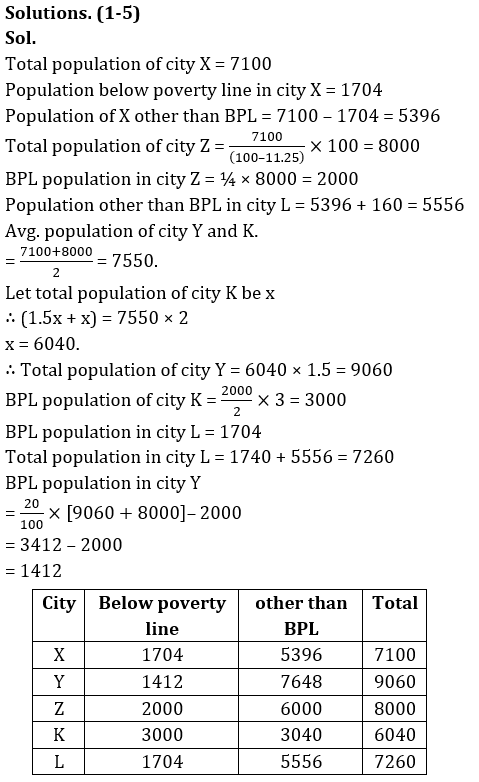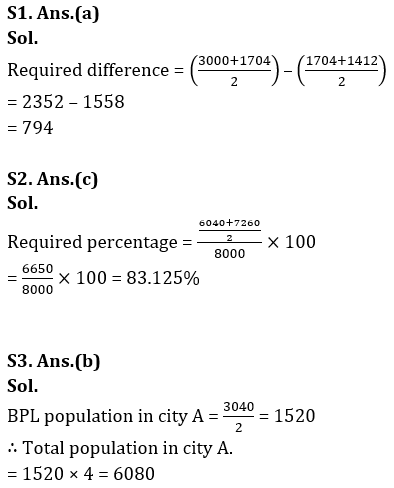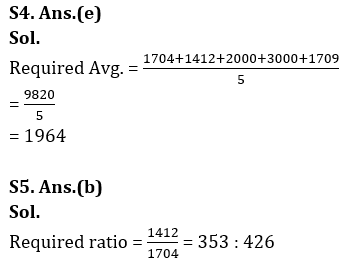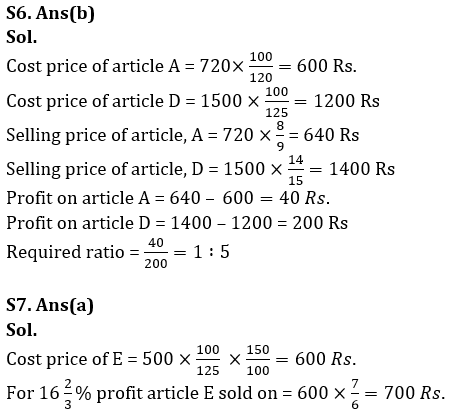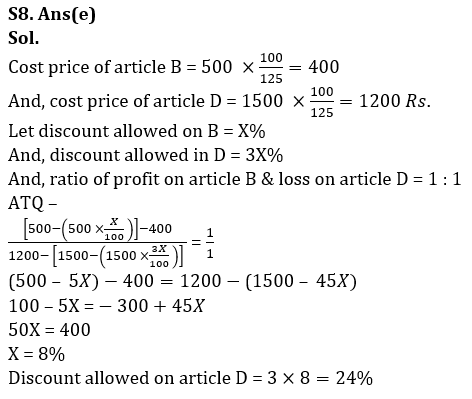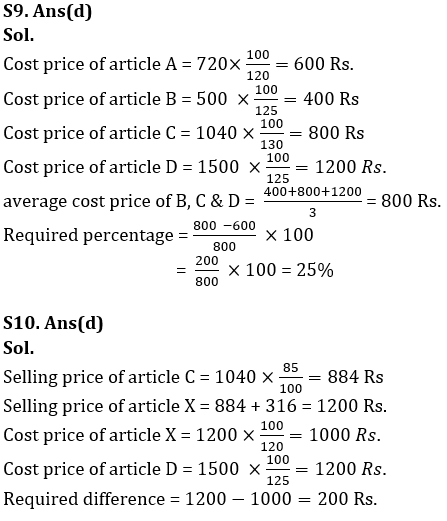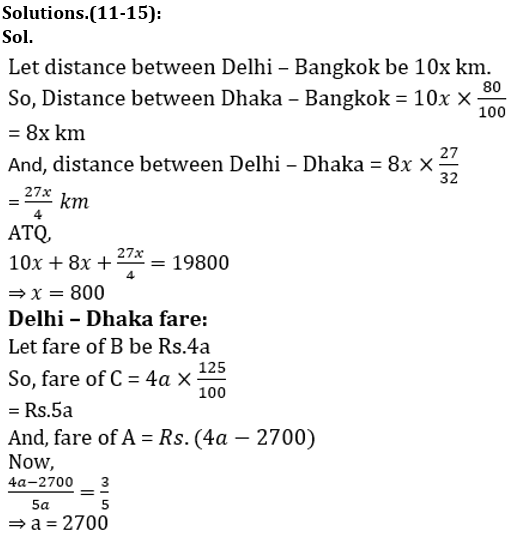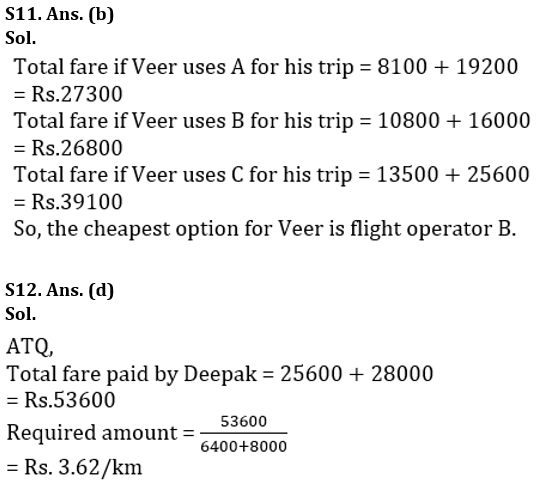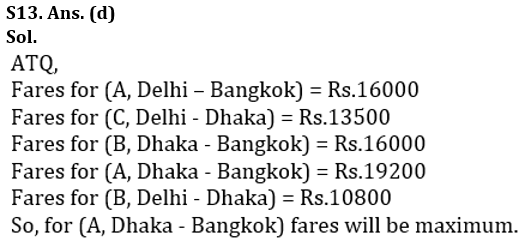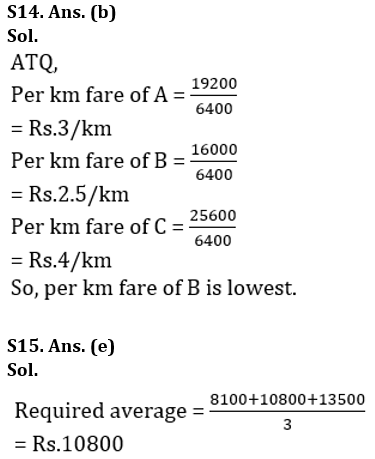## FAQs

### When is the LIC ADO Mains Exam scheduled?

The LIC ADO Mains exam is scheduled to be held on 23 April 2023

#### Congratulations!Union Budget 2023-24: Free PDF